KNOWLEDGEBASE - ARTICLE #2230

# Bug Fixed: Standard Error and 95% Confidence Interval for Y when X ≠ 0 (Deming Regression) reported incorrectly prior to Prism 9.4.1

### The big picture

With the release of Prism 9.4.1, an issue was fixed in the results of Deming Regression in which the standard error and 95% CI for reported Y values when X ≠ 0 were reported incorrectly. The results reported when X = 0 are correct in all previous versions of Prism. Additionally, this issue is not present in Prism versions prior to Prism 7.

### The details

When performing Deming Regression in Prism, the default analysis parameters will include the option to have Prism report the “95% confidence interval of Y when X = 0”. When this option is enabled, Prism will also report the associated standard error in the results sheet.

Note that by default, the value of X is set to zero, meaning that the associated Y value is the Y-intercept for the model. Importantly, when this value is set to zero, the results that Prism reported were correct. However, there was an issue in the way that standard error was calculated, resulting in standard error values that were incorrect for any other value of X. The amount of inconsistency was proportional to the distance of the X value to the origin (larger absolute values of X resulted in larger inconsistencies in the reported standard error).

Because the 95% confidence interval is determined using the calculated standard error, these values were also incorrect for any value of X other than zero (but were correct for the default X=0 case).

Results in versions prior to 9.4.1

 Standard Error and 95% CI for Y when X = 0 (Correct) Standard Error and 95% CI for Y when X ≠ 0 (Incorrect)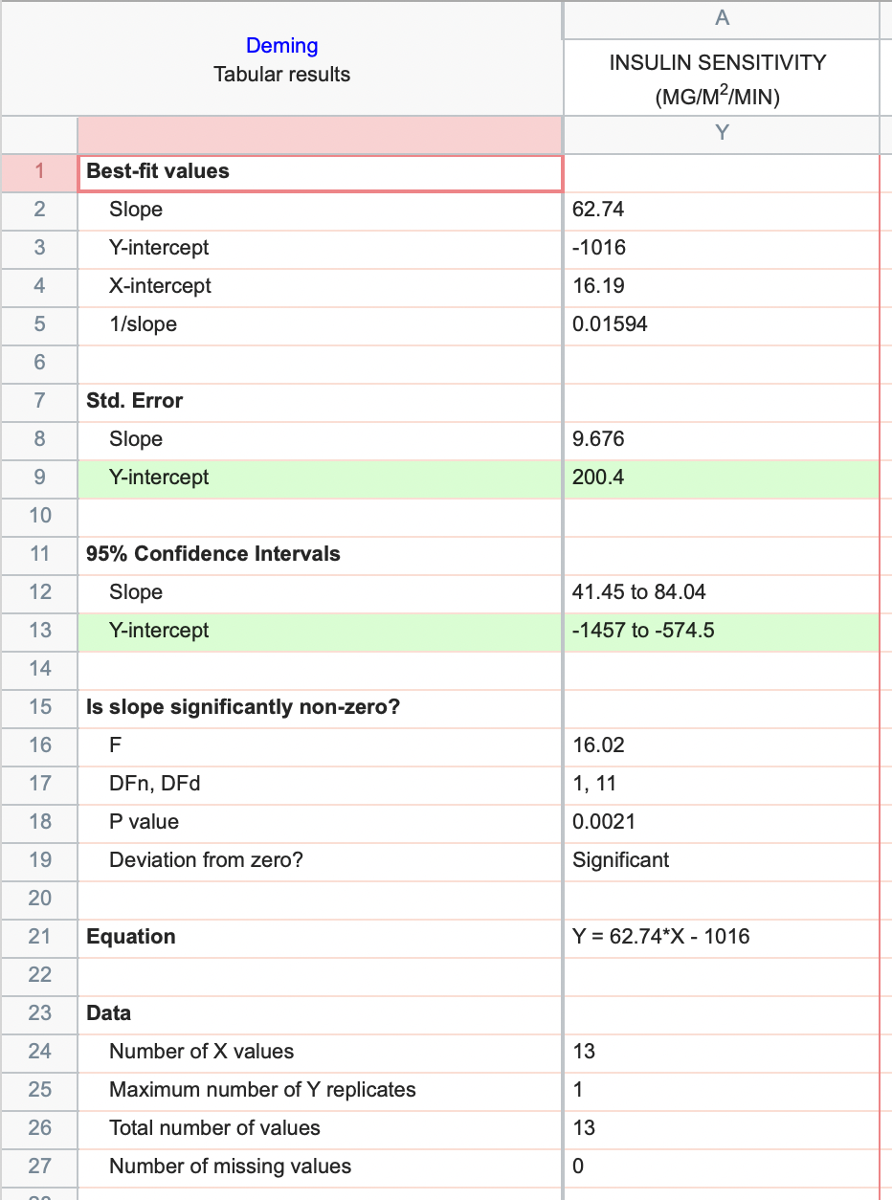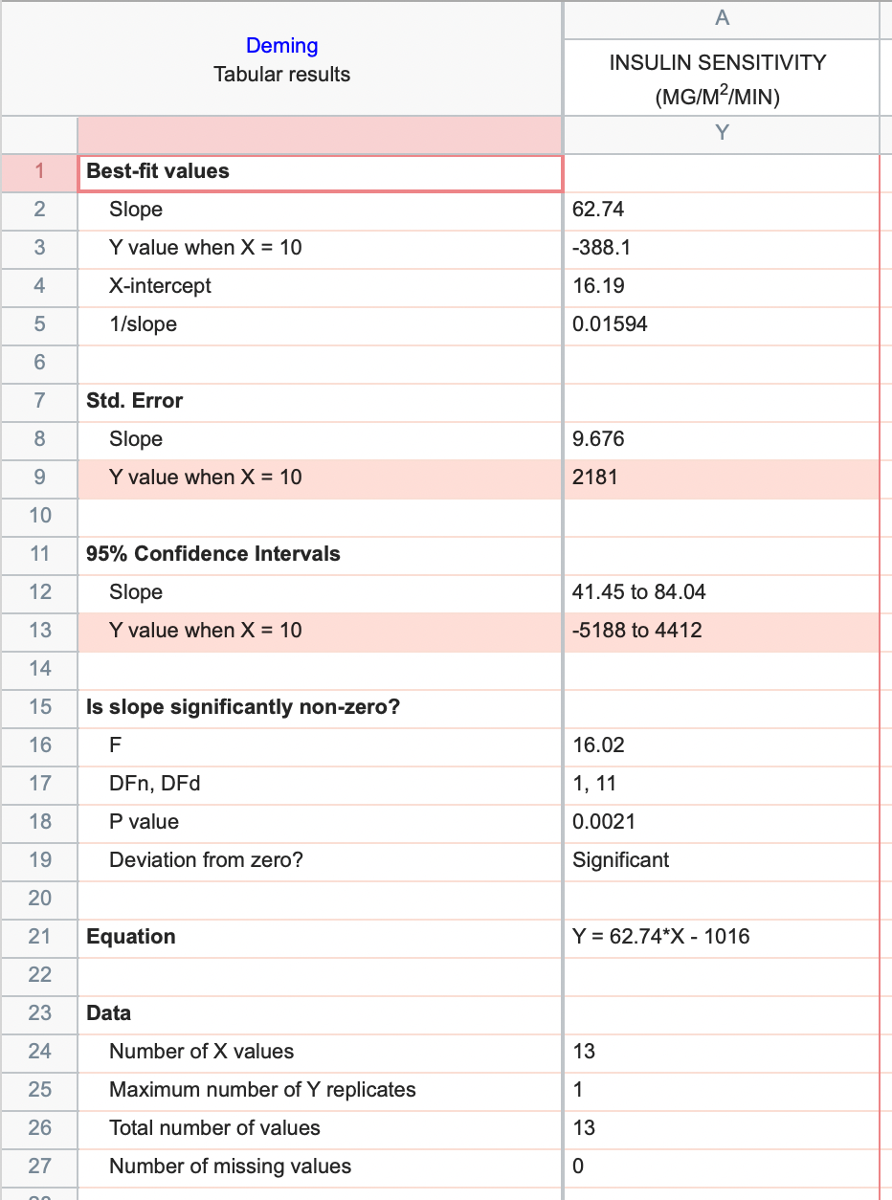Updated results beginning in version 9.4.1

 Standard Error and 95% CI for Y when X = 0 (Correct) Standard Error and 95% CI for Y when X ≠ 0 (Incorrect)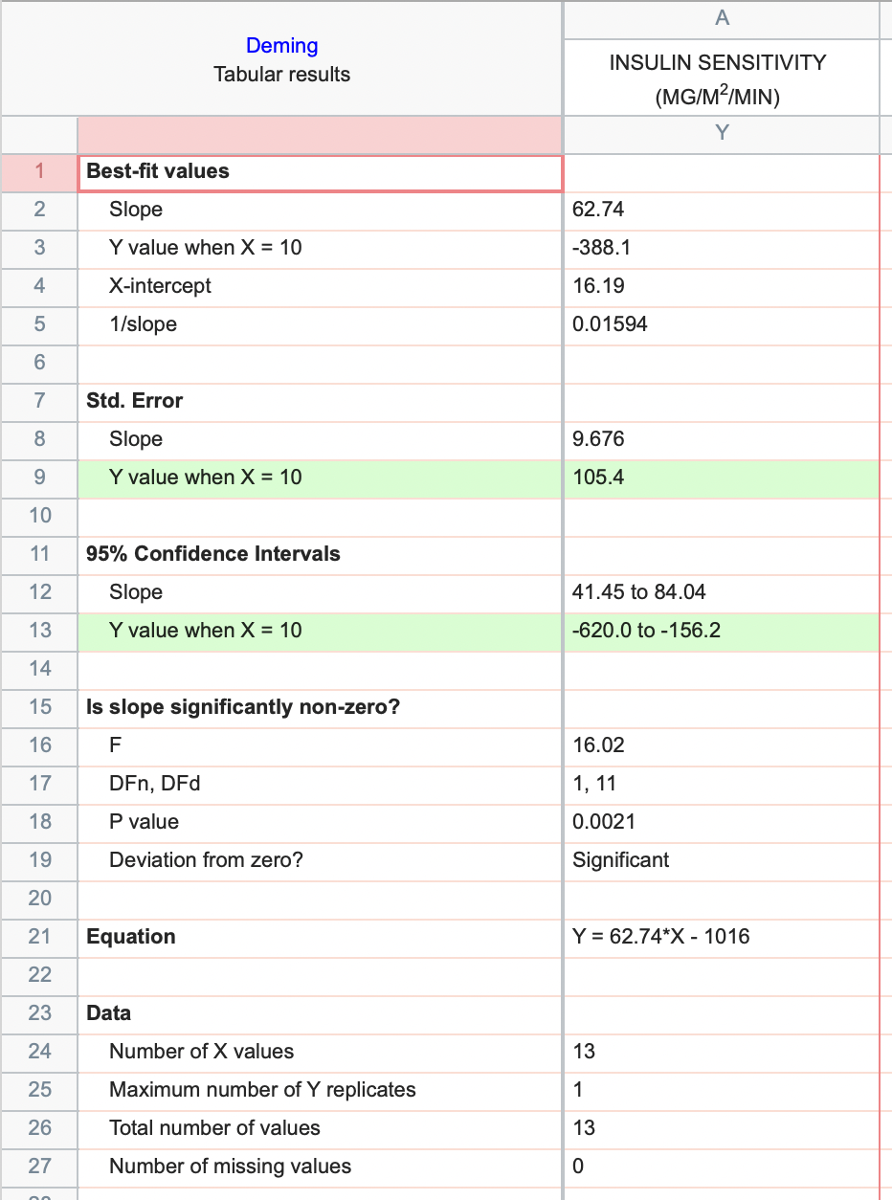### Obtaining correct Standard Error and 95% Confidence Intervals in Prism versions prior to 9.4.1

Since the standard error and 95% confidence intervals for Y are correct when X = 0, it is possible to obtain the standard error and CI for any other value of X by first subtracting this value from all X values using the Transform analysis, and then performing Deming Regression on the transformed data (keeping the default option to report standard error and 95% CI for Y when X = 0):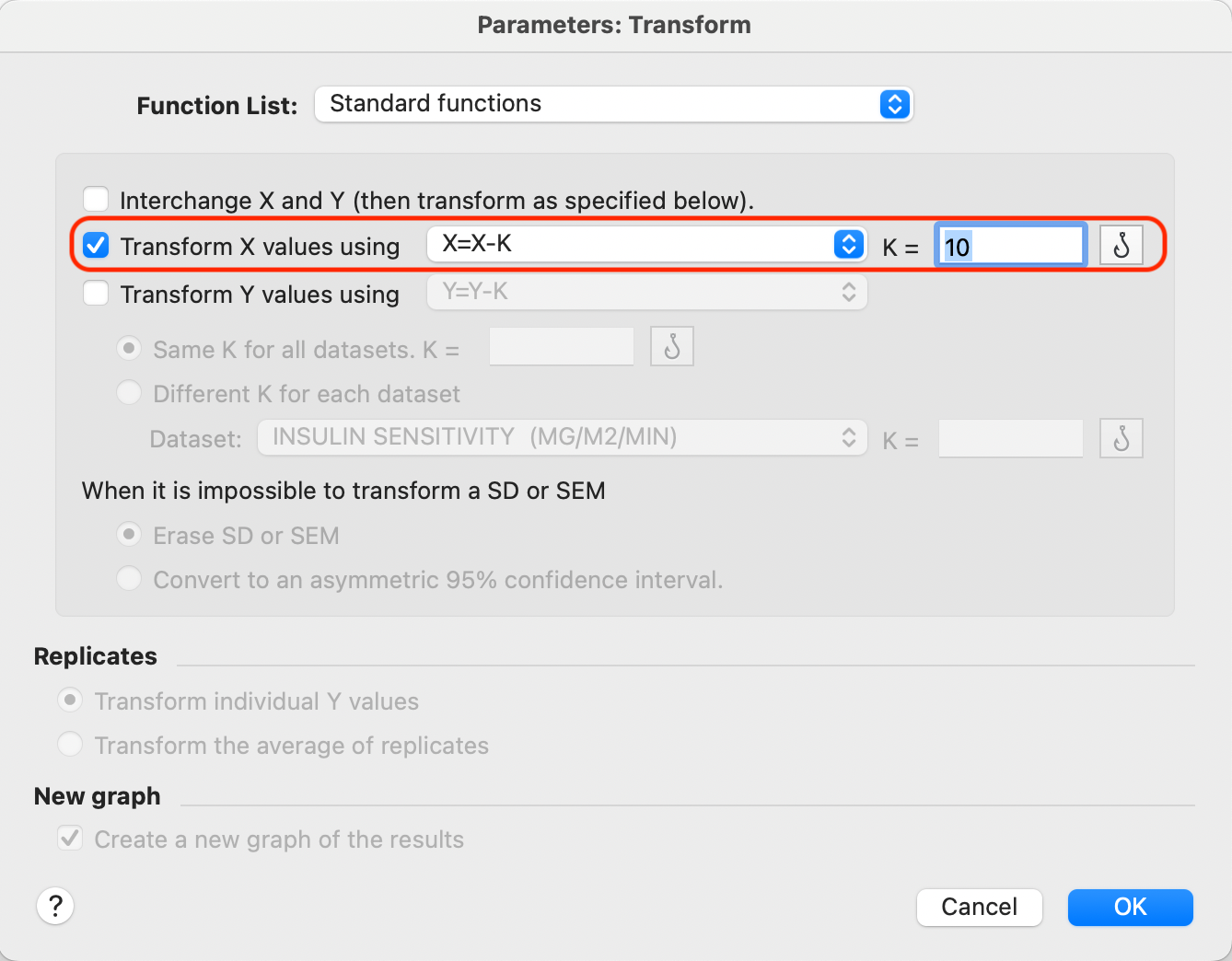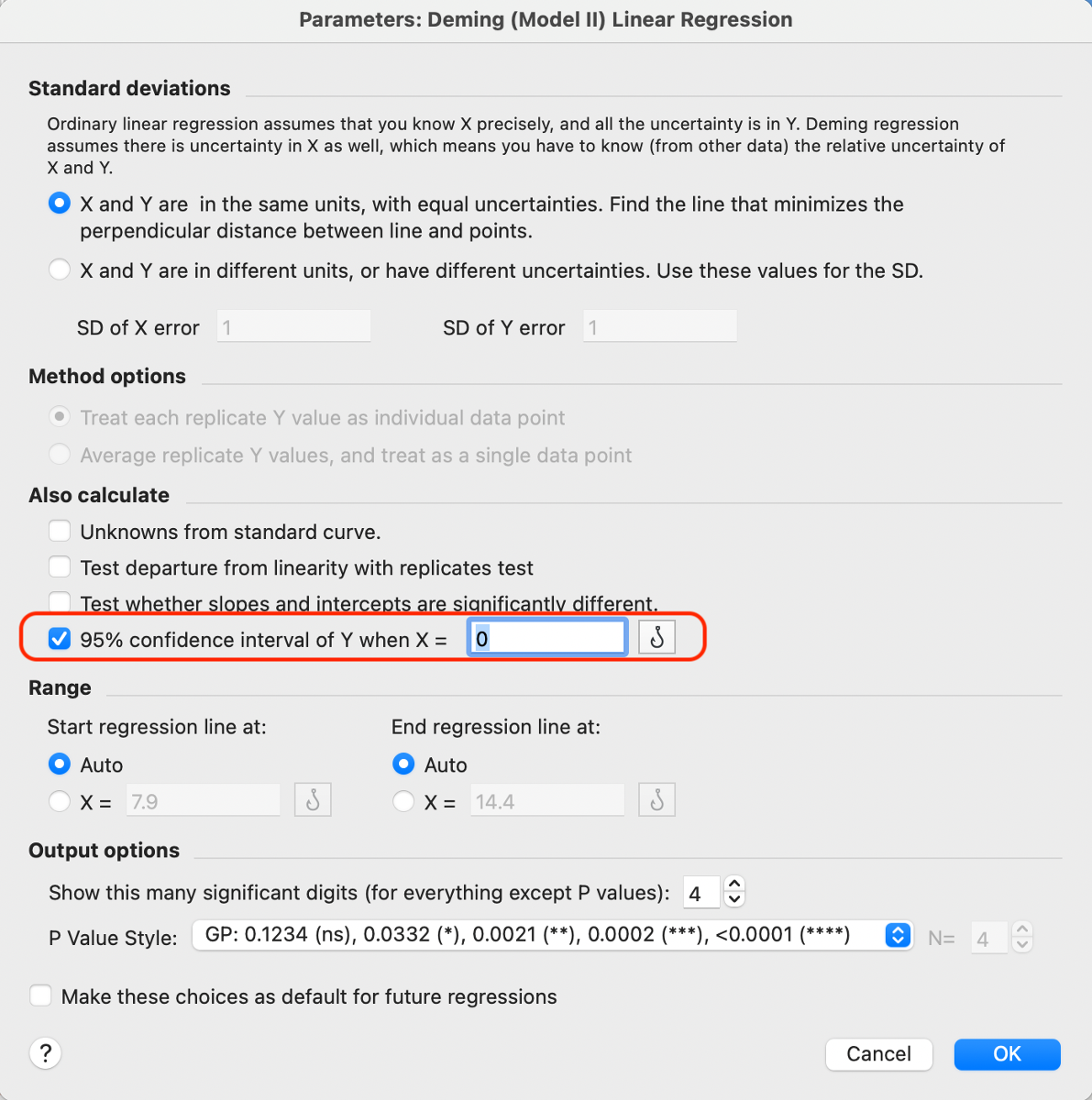If you follow these steps, the calculated standard error and 95% confidence interval will be correctly reported for the specified X value: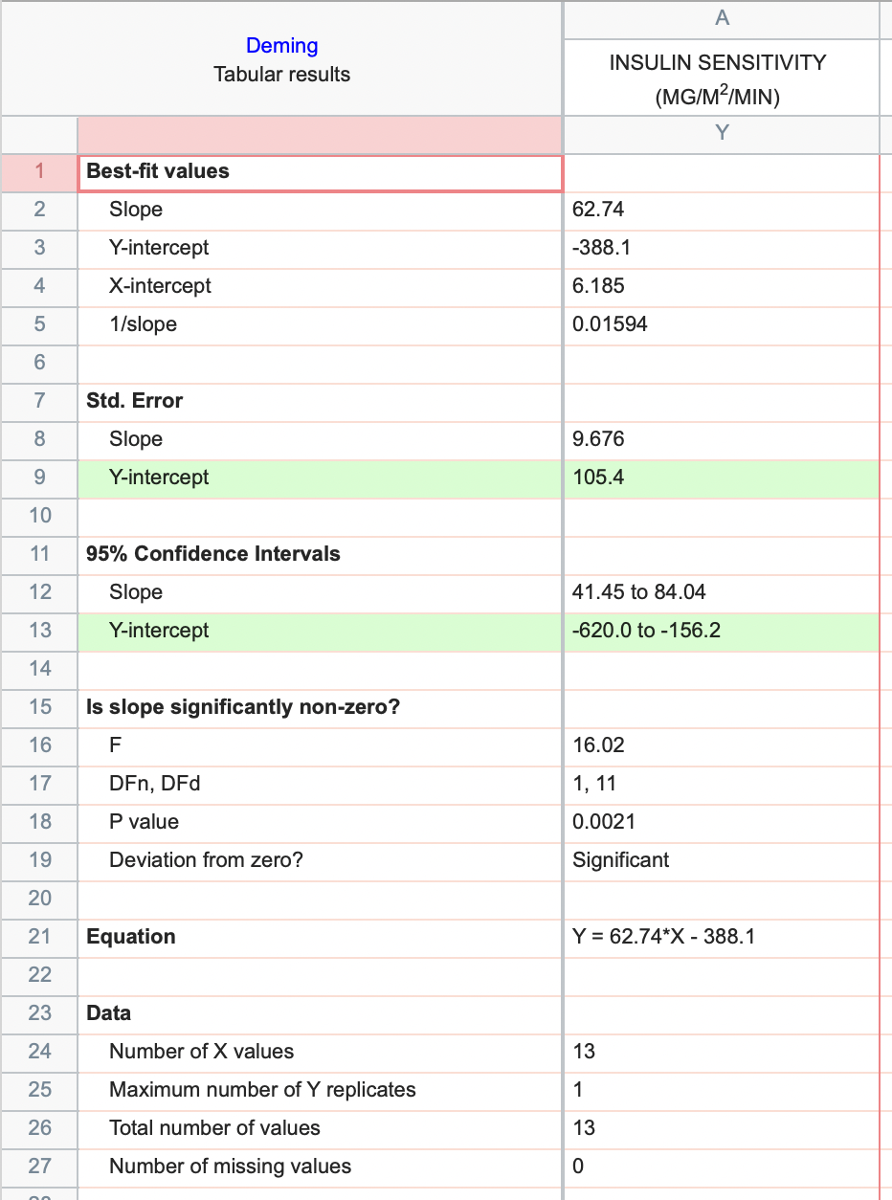## Explore the Knowledgebase

Analyze, graph and present your scientific work easily with GraphPad Prism. No coding required.